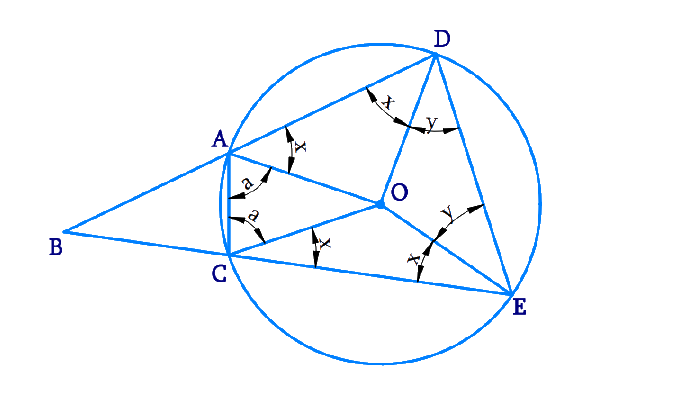# Ex.10.6 Q4 Circles Solution - NCERT Maths Class 9

## Question

Let the vertex of an angle \begin{align}{ABC}\end{align} be located outside a circle and let the sides of the angle intersect equal chords \begin{align}{AD}\end{align} and \begin{align}{CE}\end{align} with the circle. Prove that \begin{align} \angle {ABC}\end{align} is equal to half the difference of the angles subtended by the chords \begin{align}{AC}\end{align} and \begin{align}{DE}\end{align} at the centre.

Video Solution
Circles
Ex 10.6 | Question 4

## Text Solution

What is known?

Point $$B$$ lies outside the circle also $$AD$$ and $$CE$$ are equal chords.

What is unknown?

To prove that \begin{align} \angle {ABC}\end{align} is equal to half the difference of the angles subtended by the chords \begin{align} {AC}\end{align} and \begin{align}{DE}\end{align} at the centre means \begin{align}\angle ABC = \frac{1}{2}\left( {\angle DOE - \angle AOC} \right)\end{align}

Reasoning:

• The angle subtended by an arc at the centre is double the angle subtended by it at any point on the remaining part of the circle.
• A quadrilateral \begin{align}{ACED}\end{align} is called cyclic if all the four vertices of it lie on a circle.
• The sum of either pair of opposite angles of a cyclic quadrilateral is $$180^\circ.$$
• Using Side-Side-Side (SSS criteria) and Corresponding parts of congruent triangles (CPCT) we prove the statement.

Steps:Consider $$\Delta {AOD}$$ and $$\Delta {COE,}$$

\begin{align}{OA}&={OC} \qquad {\text { (Radii of the circle) }} \\{OD}&={OE} \qquad {\text { (Radii of the circle) }} \\ {AD}&={CE} \qquad {\text { (Given) }}\end{align}

$$∴ \Delta {AOD} \cong \Delta {COE}$$ (SSS congruence rule )

$$\angle {OAD}=\angle {OCE}$$ (By CPCT )  ....($$1$$

$$\angle {ODA}=\angle {OEC}$$ (By CPCT )  .....($$2$$

Also,

$$\!\angle {OAD}\!=\!\angle {ODA}$$(As $$OA=OD$$)...($$3$$)

From Equations ($$1$$), ($$2$$), and ($$3$$), we obtain

$\!\! \angle OAD \! = \!\angle OCE = \!\!\angle {ODA}= \!\! \angle {OEC}$

\begin {align} \angle {OAD} & =\angle {OCE} \\ &=\angle {ODA}\\ &=\angle {OEC}\\ &=x \end {align}

In \begin {align} \Delta {OAC,} \end {align}

\begin{align} {OA} &={OC} \\ ∴ {OCA} &=\angle {OAC}(\text { Let } a) \end{align}

In \begin {align} \Delta {ODE,} \end {align}

\begin{align} \rm{OD} &=\rm{OE} \\ \angle \rm{OED} &=\angle \rm{ODE}\text{ (Let y)} \end{align}

\begin{align}\rm{ADEC}\end{align} is a cyclic quadrilateral.

$$∴ \angle {CAD}+\angle {DEC}=180^{\circ}$$ (Opposite angles are supplementary)

\begin{align} x+a+x+y&=180^{\circ} \\ \!2 x+a+y&=180^{\circ} \\ y \!&=\!180^{\circ}-2 x \! -a ...\! (4) \end{align}

However, $$\angle {DOE}=180^{\circ}-2 {y}$$

And, \begin {align}\angle{AOC}=180^{\circ}-2 {a} \end {align}

\begin{align} & \angle {DOE}-\angle {AOC} \\&=2a-2y \\ & =2 a-2\left(180^{\circ}-2 x-a\right) \\ &=4 a+4 x-360^{\circ} \ldots \ldots (5) \end{align}

$$\angle {BAC}+\angle {CAD}=180^{\circ}$$(Linear pair )

\begin{align} ∴ \angle {BAC}&=180^{\circ}-\angle{CAD} \\ &=180^{\circ}-(a+x)\end{align}

Similarly, \begin {align} \angle {ACB}=180^{\circ}-(a+x) \end {align}

In \begin {align} \Delta {ABC,} \end {align}

$$\angle {ABC}+\angle {BAC}+\angle {ACB}=180^{\circ}$$

(Angle sum property of a triangle)

\begin {align}\angle {ABC} &=180^{\circ}-\angle {BAC}-\angle {ACB} \\ &= \begin{Bmatrix} 180^{\circ} \\ -\left(180^{\circ}-a-x\right) \\ -\left(180^{\circ}-a-x\right) \end{Bmatrix} \\ &=2 a+2 x-180^{\circ} \\ &=\frac{1}{2}\left[4 a+4 x-360^{\circ}\right]\end {align}

Using Equation ($$5$$)

\begin {align}\angle {ABC}=\frac{1}{2}[\angle {DOE}-\angle {AOC}] \end {align}

Hence it is proved that \begin{align} \angle {ABC}\end{align} is equal to half the difference of the angles subtended by the chords \begin{align}{AC}\end{align} and \begin{align}{DE}\end{align} at the centre.

Video Solution
Circles
Ex 10.6 | Question 4
Learn from the best math teachers and top your exams

• Live one on one classroom and doubt clearing
• Practice worksheets in and after class for conceptual clarity
• Personalized curriculum to keep up with school